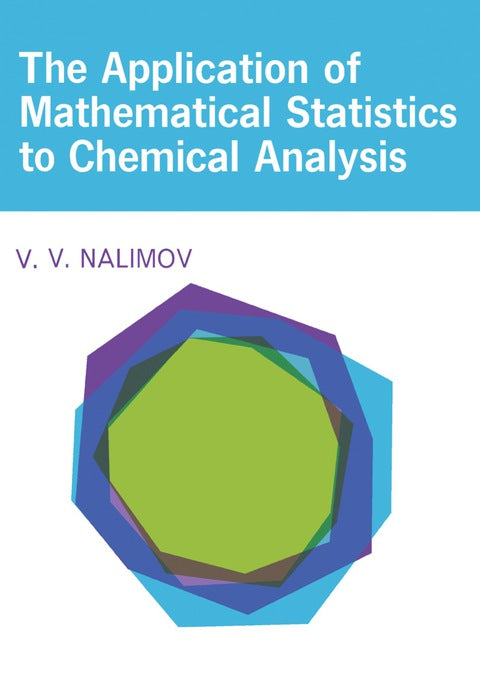• Author(s) V. V. Nalimov
• Edition
• Published05092014
• PublisherElsevier S & T
• ISBN9781483184791
The Application of Mathematical Statistics to Chemical Analysis presents the methods of mathematical
statistics as applied to problems connected with chemical analysis. This book is divided into nine chapters that particularly consider the principal theorems of mathematical statistics that are explained with examples taken from researchers associated with chemical analysis in laboratory work.

This text deals first with the problems of mathematical statistics as a means to summarize information in chemical analysis. The next chapters examine the classification of errors, random variables and their characteristics, and the normal distribution in mathematical statistics. These topics are followed by surveys of the application of Poisson's and binomial distribution in radiochemical analysis; the estimation of chemical analytic results; and the principles and application of determination of experimental variance. The last chapters explore the determination of statistical parameters of linear relations and some working methods associated with the statistical design of an experiment.

This book will be of great value to analytical chemists and mathematical statisticians.

# The Application of Mathematical Statistics to Chemical Analysis

##### Get it instantly

Zookal account needed

\$84.68 \$89.10 Save \$4.42
• Author(s) V. V. Nalimov
• Edition
• Published05092014
• PublisherElsevier S & T
• ISBN9781483184791
The Application of Mathematical Statistics to Chemical Analysis presents the methods of mathematical
statistics as applied to problems connected with chemical analysis. This book is divided into nine chapters that particularly consider the principal theorems of mathematical statistics that are explained with examples taken from researchers associated with chemical analysis in laboratory work.

This text deals first with the problems of mathematical statistics as a means to summarize information in chemical analysis. The next chapters examine the classification of errors, random variables and their characteristics, and the normal distribution in mathematical statistics. These topics are followed by surveys of the application of Poisson's and binomial distribution in radiochemical analysis; the estimation of chemical analytic results; and the principles and application of determination of experimental variance. The last chapters explore the determination of statistical parameters of linear relations and some working methods associated with the statistical design of an experiment.

This book will be of great value to analytical chemists and mathematical statisticians.